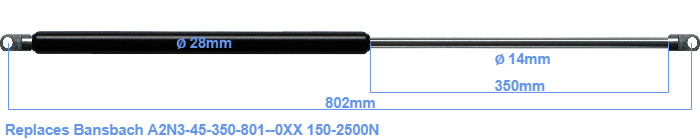# Replacement for Bansbach A2N3-45-350-801–0XX 150-2500N

\$56.36

Replacement gas spring for the Bansbach A2N3-45-350-801--0XX 150-2500 Newton. The eye has a thickness of 10mm and a hole diameter of 8.1mm. Brand: HAHN, a Stabilus company.

Please note:
• Mounting part tube: The thickness of the eye of this replacement is 10mm instead of 8mm.
• Mounting part rod : The thickness of the eye of this replacement is 10mm instead of 18mm.
•  Force Choose an option150 Newton200 Newton250 Newton300 Newton350 Newton400 Newton450 Newton500 Newton550 Newton600 Newton650 Newton700 Newton750 Newton800 Newton850 Newton900 Newton950 Newton1000 Newton1050 Newton1100 Newton1150 Newton1200 Newton1250 Newton1300 Newton1350 Newton1400 Newton1450 Newton1500 Newton1550 Newton1600 Newton1650 Newton1700 Newton1750 Newton1800 Newton1850 Newton1900 Newton1950 Newton2000 Newton2050 Newton2100 Newton2150 Newton2200 Newton2250 Newton2300 Newton2350 Newton2400 Newton2450 Newton2500 NewtonClearThis gas spring has a cylinder diameter of 28 mm. The diameter of the rod totals 14 mm. The rod (the chrome part) is 350 mm long. The total length of this gas strut is 802 mm. This is the total distance between the centers of the two mounting parts. The length from thread to thread (exlcuding the mounting parts) is 748 millimeter. The force of this replacement is 150-2500 Newton. This gas spring can replace a Bansbach gas spring, but is not an orignal Bansbach one. HAHN Gasfedern, a Stabilus subsidiary, is responsible for the production of this replacement gas spring. The gas spring has the brand HAHN. Nevertheless, the dimensions and force are close to equal.